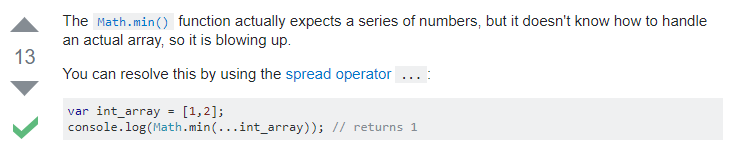# I need help with a JavaScript challenge on Checkio

## Recommended Posts

Hey everybody, I'm a beginner at JavaScript so there are many things I don't understand. I'd appreciate very much if you can help me figuring out something new.

I'm trying to solve this problem on Checkio.org called "The Most Numbers". The requirement is quite simple: It is given an array of floats and you have to calculate the difference between the maximum and minimum numbers. And this is my solution (which didn't work):

```function mostNumbers(numbers){
if (numbers.length > 0) {
return Math.max(numbers) - Math.min(numbers);
} else {
return 0;}
}```

It produced "0" regardless of what "numbers" is.

I tried some stuff related to the Math.max() and Math.min() methods. And this is what I received:

`Math.max(1,2,3) // => 3`

But when I assigned the array to a variable, this is what I received:

```var numbers = (1,2,3);
Math.max(numbers) // => 3 (nothing wrong)
Math.min(numbers) // => 3 (why?)```

I realized that it always take the last number, regardless what I want, so I tried typing (1,2,3) into the console and I got 3. Why is that? Then I realized that this is not a normal array, which has the structure [something, something else,...].

I reached out to w3school but what I received are just simple examples like

`Math.max(5, 10); // => 10`

I reached out to stackoverflows as well and found this:With all the respect for this person (who is contributing value, that's great), I find the problem even more confusing.

- What is a series of numbers, if not an array of numbers?

- What is a spread operator? I clicked on the link he/she provided, but I didn't understand what I read:

Quote

Spread syntax allows an iterable such as an array expression or string to be expanded in places where zero or more arguments (for function calls) or elements (for array literals) are expected, or an object expression to be expanded in places where zero or more key-value pairs (for object literals) are expected. (https://developer.mozilla.org/en-US/docs/Web/JavaScript/Reference/Operators/Spread_operator)

Thank you for reading my post, I know it's long. I appreciate it.

Edited by BaoDanh
##### Share on other sites

9 hours ago, BaoDanh said:

if (numbers.length > 0) {

.. The problem seems to me is in this statement. Not sure if 'length' is the property you are looking for.  I believe that is used to find how elements you have in an array. Wouldn't it be more like:

`if(numbers > 0) { }`

Stef

##### Share on other sites

I think the first if statement is correct, Stefan, since we must be sure that the numbers array actually contains at least one number. So, numbers.length must indeed be strictly greater than 0.

What is not correct is...

1) The initial variable declaration:

```var numbers = (1,2,3); // You can't use parentheses to declare an array without using the array keyword like this:
//var numbers = new Array(1,2,3);

// Instead, you have to declare an array by using the short square brackets notation:
var numbers = [1,2,3];```

2) The arguments passed to the max() and the min() methods of the Math object can only be a list of numbers separated by commas, but not an array of numbers.
So, to use it on an array, we first need to reduce this array...

```// ... a first time to get the maximum value:
var maxValue = numbers.reduce(function(a, b) {
return Math.max(a, b);
});

// ... a second time to get the minimum value:
var minValue = numbers.reduce(function(a, b) {
return Math.min(a, b);
});```

And only then you substract the two previously obtained values.

`var differenceValue = maxValue - minValue;`

You function becomes:

` `

The text editor doesn't allow me to write the overall body of the mostNumbers() function, so I attached a text file to my reply for that.

`mostNumbers(numbers);  // We call the function to test it in the browser.`

(Note: in addition to the initial if statement, we should check that all the values included in the numbers array only contain actual numbers, and otherwise display a message that warns that at least one member of the array is not a number.)

Edited by Kwisatsoundman

## Join the conversation

You can post now and register later. If you have an account, sign in now to post with your account.
Note: Your post will require moderator approval before it will be visible.×   Pasted as rich text.   Paste as plain text instead

Only 75 emoji are allowed.

×   Your previous content has been restored.   Clear editor

×   You cannot paste images directly. Upload or insert images from URL.